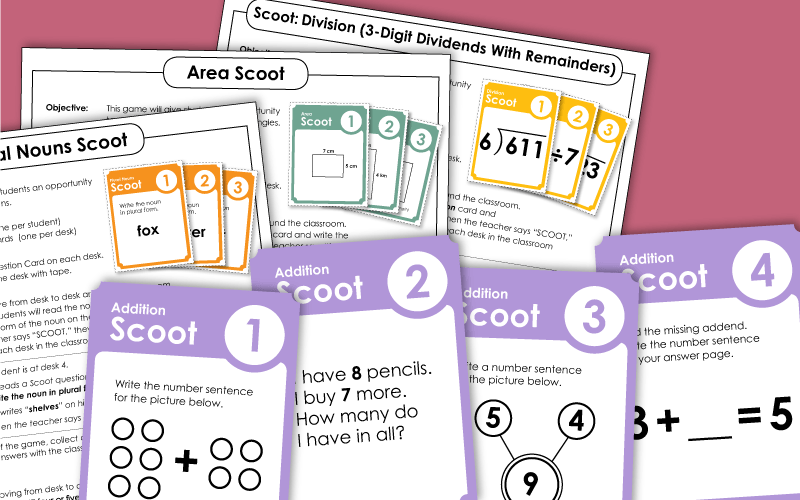# Scoot Classroom Game

Scoot! is a whole-class educational game that your students will love. Place a question card on each desk in your classroom. To play, students move around the room, from desk to desk, answering questions or solving math problems.## Math

This fun math game has 30 question cards, an instruction sheet, and grid answer sheets for 20, 25, and 30 questions. The sums in these addition problems are 10 and under.
Kindergarten to 2nd Grade
In this interactive, whole-class game, students move to each desk in the classroom to answer basic addition questions. This version of the game includes simple facts with sums up to 20.
Kindergarten to 2nd Grade
This game can be used to help students practice 2-digit addition. Most problems require carrying/regrouping. (example: 83+22)
1st and 2nd Grades
This version of the game has thirty task cards with three-digit addition problems. (example: 492+183)
2nd through 4th Grades
Students calculate the area of the given squares and rectangles. Requires only basic multiplication skills. All units are metric.
Solve division problems with remainders. Each problem has a 3-digit dividend and a 1-digit divisor.
3rd and 4th Grades
Solve long division problems with 4-digit dividends and 1-digit divisors. Most of the problems on these task cards have remainders.
Students write the correct fraction shown on each of the 30 task cards.
2nd through 4th Grades
The Multiplication Scoot! game includes arrays, number bonds, and missing factor problems. This game has 30 question cards, teacher instructions, and student answer worksheets.
3rd and 4th Grades
Pupils multiply three-digit numbers by single-digit numbers.
3rd through 6th Grades
In this version of the game, students multiply by 2-digit numbers. Includes horizontal and vertical problems. (example: 583x74)
4th through 6th Grades
This version of the game has 30 cards. Each card has a polygon with 3, 4, 5, or 6 sides. Students add to calculate the perimeter of each polygon.
3rd through 5th Grades
This game has cards with 5-digit place value questions. The find the value of an underlined digit, compare numbers, recognize number
3rd and 4th Grades
Each card has a two-digit number on it. Round the numbers shown to the nearest ten. This version only has 2-digit numbers.
(example: Round 24 to the nearest ten.)
2nd through 4th Grades
Round each number to the nearest ten. This version has a mix of 2 and 3-digit numbers.
3rd and 4th Grades
These Scoot cards have three-digit numbers on them. Practice rounding to the nearest hundred. This version has 3-digit numbers only.
2nd through 4th Grades
Round the numbers shown to the nearest hundred. This version of Scoot! includes cards with 3-digit and 4-digit numbers.
3rd and 4th Grades
This Scoot! game has math problems with 3-digit subtraction.

## EnglishLanguage Arts

Contractions  FREE
Each task card has a pair of words. Students write the correct contraction for each.
1st through 4th Grades
Write the plural form of the nouns on the task cards. Includes regular and irregular plurals. (examples: knife-knives; dish-dishes; ski-skis)
2nd through 4th Grades
For each card, students will choose two words that are synonyms or antonyms.
2nd through 5th Grades
Rewrite each sentence with an alternate verb tense. The sentences on these cards have regular and irregular verbs.
2nd through 4th Grades

## Science

This game has thirty task cards with questions about the moon, the planets, and the sun.
3rd through 6th Grades

## Templates

This template includes 30 blank Scoot! cards that you can use to write your own questions or math problems.
This file has a set of thirty red Scoot! cards. These are blank templates upon which you can write your own questions or math problems.
In this PDF, you'll find 30 blank, green cards.
This blank template includes 30 pink Scoot! card.
This file has a blank, 30-square student answer grid.
This PDF contains twenty-five empty answer boxes.
Students can write answers to Scoot! task cards on this twenty-five square grid.

These printable task cards can easily be adapted for playing Scoot! games.

"I have...Who has..." Card Games

I have-Who has is a whole-class interactive card game. This activity can be used to teach math skills, sight words, and phonics.

Classroom Scavenger Hunts

Hide clues around the classroom for students to find. Students use the clues to answer science, math, and grammar questions.

My Account
Site Information